This is a writeup of my first assignment for the “Computer Vision II: Applications” course.

For this assignment, I’m given an image of a girl, and I have to add 2 new features to it:

1. Apply lipstick
2. Applying blush

Here’s the original image that we’ll be working on: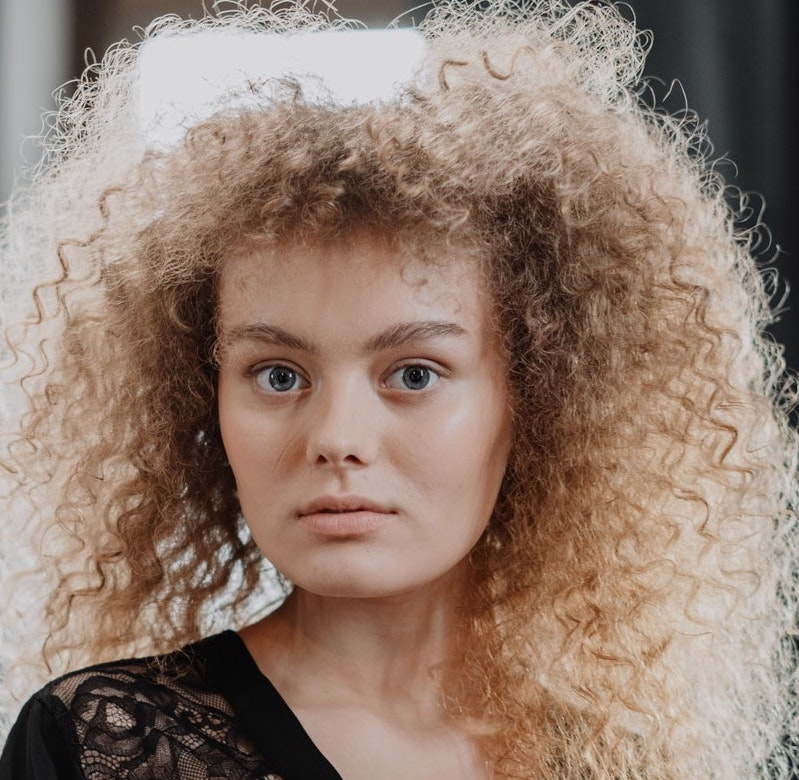## Initialisation actions#

We first load and initialise all the necessary modules and objects that we’ll need

 ``````1 2 3 4 5 6 7 8 `````` `````` # various imports import cv2, sys, dlib, time, math import numpy as np import matplotlib.pyplot as plt from pathlib import Path from typing import Tuple from cv_helpers import * from helpers import *``````

Note that `cv_helpers` and `helpers` are personal modules, mainly for debugging / visualisation purposes.

We then load the dlib 68 point face detector:

 ``````1 2 3 4 `````` `````` path = Path(__file__).absolute().parent predictor_path = path / "shape_predictor_68_face_landmarks.dat" faceDetector = dlib.get_frontal_face_detector() landmarkDetector = dlib.shape_predictor(str(predictor_path))``````

Notice that we’re taking the absolute path to the trained predictor; That’s the correct way of opening files since this allows you to run the application regardless your working directory. We then load the image via the `cv2.imread` function, taking care of the BGR -> RGB conversion since OpenCV uses the `BGR` format and finally we detect the face landmarks:

 ``````1 2 3 `````` `````` im = cv2.imread(str(path / "girl-no-makeup.jpg")) imDlib = cv2.cvtColor(im, cv2.COLOR_BGR2RGB) landmarks = fbc.getLandmarks(faceDetector, landmarkDetector, im)``````

To verify that we’ve loaded the image and we’ve detected the required features succesfully, we can plot the original image, along with it superimposed by the detected features. To do that, we’ll make use of the following helper functions:

 `````` 1 2 3 4 5 6 7 8 9 10 11 12 13 14 15 16 17 18 19 20 21 22 23 24 25 26 27 28 29 30 31 32 33 34 35 36 37 38 39 40 41 42 43 44 45 46 47 48 49 50 51 52 53 54 55 56 57 `````` `````` def mark_points_in_img(img: np.ndarray, points, inplace=True) -> np.ndarray: """ Each one of the points in the `points` sequence is marked by a circle and its corresponding index in text. """ if not inplace: img = img.copy() for i, la in enumerate(points): cv2.circle(img, la, radius=2, color=(0, 255, 0)) cv2.putText(img, str(i), la, cv2.FONT_HERSHEY_SIMPLEX, 0.5, (0, 0, 255), 1) return img def get_num_rows_cols(size: int, max_cols: int = 2) -> Tuple[int, int]: """Format `size` elements into an xy grid. >>> get_num_rows_cols(2, max_cols=4) (1, 2) >>> get_num_rows_cols(4, max_cols=4) (1, 4) >>> get_num_rows_cols(5, max_cols=4) (2, 4) >>> get_num_rows_cols(10, max_cols=4) (3, 4) >>> get_num_rows_cols(6, max_cols=2) (3, 2) """ ncols = min(size, max_cols) if size % ncols == 0: nrows = size // ncols else: nrows = int(np.ceil(size / ncols)) return nrows, ncols def plt_imshow(*imgs, is_bgr=False): """ Render the given images in matplotlib. The function will automatically arrange them in a grid layout if they are more than 2. """ if is_bgr: imgs = [cv2.cvtColor(img, cv2.COLOR_BGR2RGB) for img in imgs] if not imgs: return n = len(imgs) rows, cols = get_num_rows_cols(n, max_cols=3) fig = plt.figure(figsize=(8, 8)) for i, img in enumerate(imgs): fig.add_subplot(rows, cols, i + 1) plt.imshow(img) plt.show()``````

And now to put these functions to good use:

 ``````1 2 3 `````` `````` # Copy the image - keep the original intact im1 = mark_points_in_img(imDlib, landmarks, inplace=False) plt_imshow(imDlib, im1)``````

And here’s the result: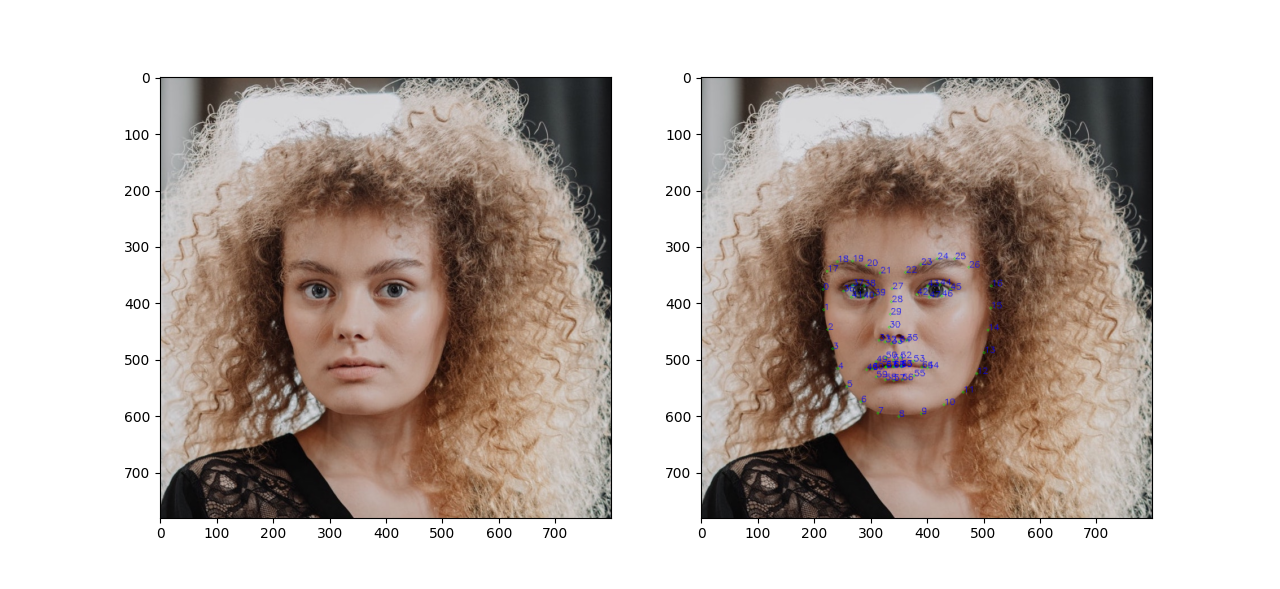## Applying lipstick#

To apply lipstick to the girl in the image, we follow the steps below:

1. Identify the list of landmarks that comprise the lips area as well as the area that corresponds to the mouth cavity (in case the person has slightly opened their mouth).
2. Initialise a new black image, that has the same size as the original one, and has a solid red color at the lips.
3. Slightly blur the latter, in order to soften its edges and hide potential defects in the end result
4. Finally do an alpha-blend of the two images (original girl image, lipsticks only image) giving a higher weight to the girl
 `````` 1 2 3 4 5 6 7 8 9 10 11 12 13 14 15 16 17 18 19 20 21 22 23 24 `````` `````` im_lipstick = im.copy() # gather the indexes of the outer and inner lip cavities lips_outer_idx = range(48, 60) lips_outer_landmarks = [landmarks[i] for i in lips_outer_idx] lips_inner_idx = range(60, 67) lips_inner_landmarks = [landmarks[i] for i in lips_inner_idx] # create lipstick-only area im_lipstick_only = np.zeros(im_lipstick.shape, dtype=im_lipstick.dtype) cv2.fillPoly( im_lipstick_only, np.array([lips_outer_landmarks], np.int32), color=(0, 0, 255), ) cv2.fillConvexPoly( # mouth cavity im_lipstick_only, np.array(lips_inner_landmarks, np.int32), color=(0, 0, 0), ) # do a minor blur to sotften the edges im_lipstick_only = cv2.blur(im_lipstick_only, (5, 5)) alpha = 0.8 im_lipstick = cv2.addWeighted(im, alpha, im_lipstick_only, 1 - alpha, 0.0) plt_imshow(im, im_lipstick, is_bgr=True)``````

Here’s how the girl looks after having applied the lipstick: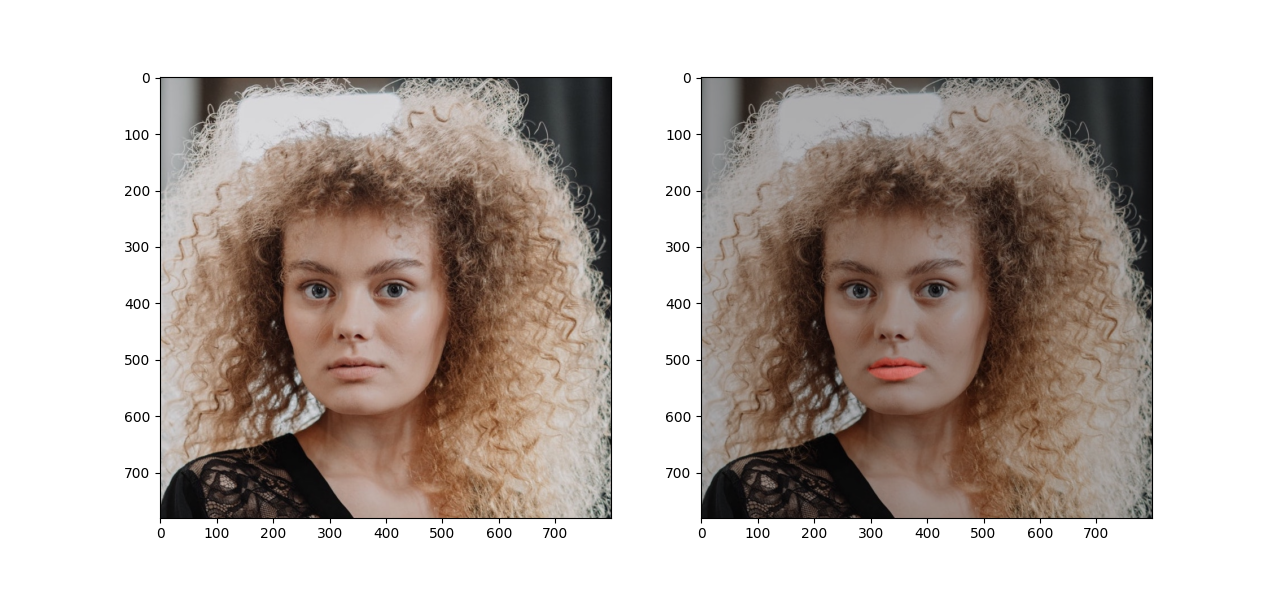## Applying blush#

Let’s start where we left off at the previous section,

 ``````1 2 `````` `````` im = im_lipstick im = cv2.cvtColor(im, cv2.COLOR_BGR2RGB)``````

Our goal is to use a second image, let’s call it `im2` which has intense blush characteristics, and try to somehow blend it with our original image, `im`. To do that, we first normalise both `im` and `im2` to a specifc size and using specific features and distances as reference for the normalisation. For this, as in the first step, we will use the `fbc.normalizeImagesAndLandmarks` function provided in the course material. However, instead of using the corners of the eyes, as the points for normalisation , we will instead use the distance between the cheek bones. Thus we add an extra class to encode this extra information, and we change the signature of the `fbc.normalizeImagesAndLandmarks` function as follows:

 `````` 1 2 3 4 5 6 7 8 9 10 11 12 13 14 15 16 17 18 19 20 21 22 `````` `````` class PointsForNormalisation: loc0: Tuple[int, int] = (0, 0) loc1: Tuple[int, int] = (0, 0) new_loc0: Tuple[int, int] = (0, 0) new_loc1: Tuple[int, int] = (0, 0) def normalizeImagesAndLandmarks(outSize: Tuple[int, int], imIn, pointsIn: np.ndarray, points_for_normalisation: Optional[PointsForNormalisation]=None): ... if points_for_normalisation: ps = points_for_normalisation loc0 = ps.loc0 loc1 = ps.loc1 new_loc0 = ps.new_loc0 new_loc1 = ps.new_loc1 else: # business as usual # use corners of eyes``````

We then execute the normalisation as follows:

 `````` 1 2 3 4 5 6 7 8 9 10 11 12 13 14 15 16 17 18 19 20 21 22 23 24 25 26 27 28 29 30 31 `````` `````` dst_shape = (600, 600) landmarks_arr = np.array(landmarks) def get_ps_for_cheeks(landmarks: np.ndarray, w, h) -> fbc.PointsForNormalisation: ps = fbc.PointsForNormalisation() ps.loc0 = landmarks ps.loc1 = landmarks ps.new_loc0 = (np.int(0.15 * w), np.int(h / 2)) ps.new_loc1 = (np.int(0.85 * w), np.int(h / 2)) return ps im, landmarks_arr = fbc.normalizeImagesAndLandmarks(dst_shape, im, landmarks_arr, get_ps_for_cheeks(landmarks_arr, dst_shape, dst_shape)) landmarks = [(la, la) for la in landmarks_arr] assert im.shape[:-1] == dst_shape # load, find landmarks in and reshape second image im2_orig = cv2.imread(str(path / "makeup2.jpeg")) im2 = cv2.cvtColor(im2, cv2.COLOR_BGR2RGB) landmarks2 = fbc.getLandmarks(faceDetector, landmarkDetector, im2) landmarks_arr2 = np.array(landmarks2) im2, landmarks_arr2 = fbc.normalizeImagesAndLandmarks(dst_shape, im2, landmarks_arr2, get_ps_for_cheeks(landmarks_arr2, dst_shape, dst_shape)) landmarks2 = [(la, la) for la in landmarks_arr2] assert im2.shape[:-1] == dst_shape``````

Let’s verify that the normalisation works as expected:

 ``````1 2 3 4 5 6 `````` `````` plt_imshow( cv2.cvtColor(im_lipstick, cv2.COLOR_BGR2RGB), cv2.cvtColor(im2_orig, cv2.COLOR_BGR2RGB), im, im2, )``````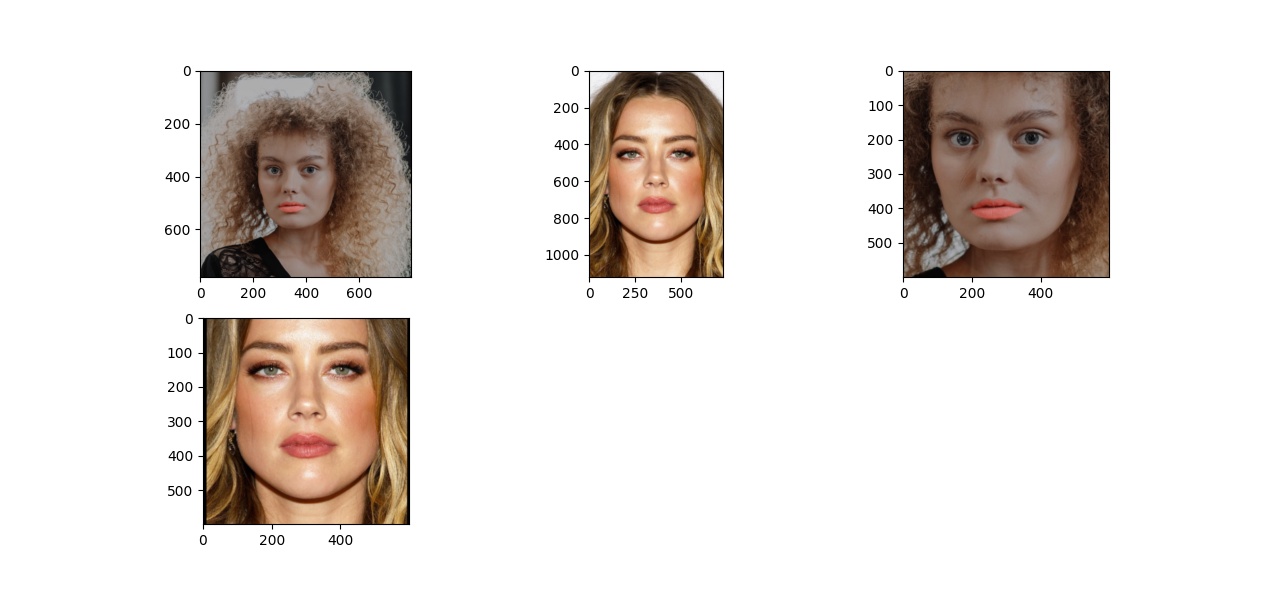Initially I thought that doing delaunay triangulation and then warping the triangles around the cheeks area would solve it, however, as demonstrated in the course materials, because of the different texture and skin color, this doesn’t work and the end result doesn’t look good. I then decided to opt for two seamless cloning operations, one for each cheek, and circular patches in the cheeks as the mask.

Here’s how I finally implemented it:

 `````` 1 2 3 4 5 6 7 8 9 10 11 12 13 14 15 16 17 18 19 20 21 22 23 24 25 26 27 28 `````` `````` # Find the center of each cheek - that's where the kernel of the blush will be def get_cheek_loc(a: tuple, b: tuple): # return (a + b)//2, (a + b)//2 xdist = b - a ydist = b - a x = int(a + 1 / 3 * xdist) y = int(a + 1 / 3 * ydist) return x, y cheek1_xy = get_cheek_loc(landmarks, landmarks) cheek2_xy = get_cheek_loc(landmarks, landmarks) # create the masks mask1 = np.zeros(im.shape, im.dtype) mask2 = mask1.copy() cv2.circle(mask1, cheek1_xy, radius=50, color=(255, 255, 255), thickness=cv2.FILLED) cv2.circle(mask2, cheek2_xy, radius=50, color=(255, 255, 255), thickness=cv2.FILLED) # create a copy for visualisation purposes - show where we copied pixels from im2_ = im2.copy() cv2.circle(im2_, cheek1_xy, radius=50, color=(255, 255, 255)) cv2.circle(im2_, cheek2_xy, radius=50, color=(255, 255, 255)) dst = cv2.seamlessClone(np.uint8(im2), im, mask1, cheek1_xy, cv2.NORMAL_CLONE) dst = cv2.seamlessClone(np.uint8(im2), dst, mask2, cheek2_xy, cv2.NORMAL_CLONE) plt_imshow(im, im2_, dst)``````

Here’s how the final image looks like: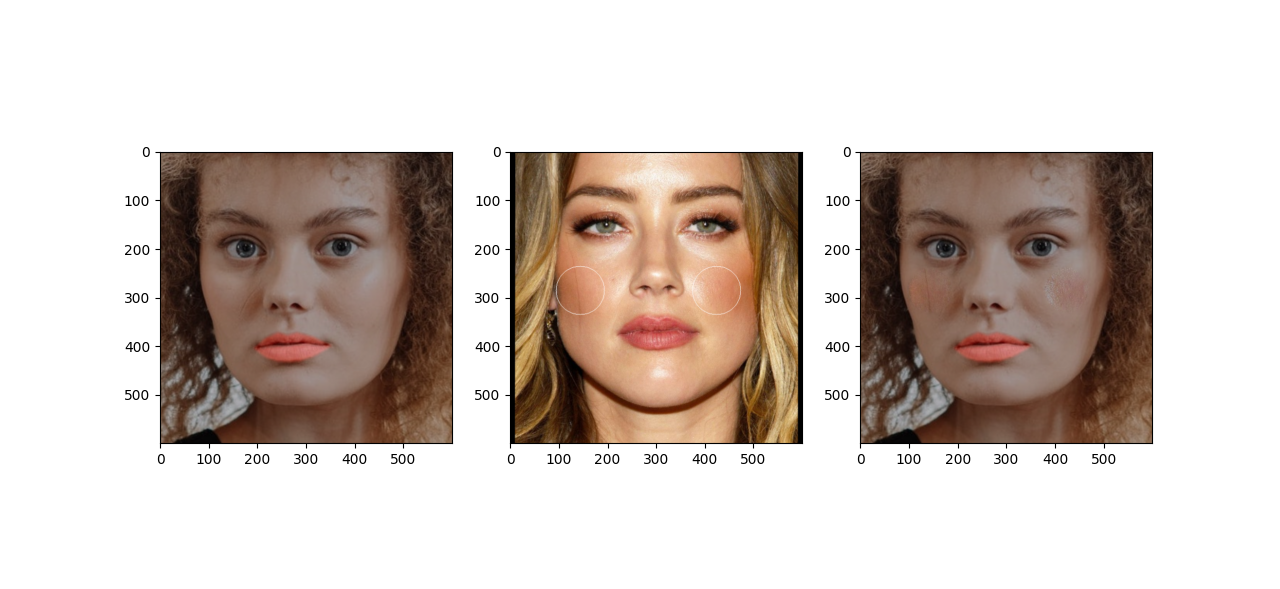As you can see, the seamless cloning operations brought a few inaccuracies from `im2` however the blush itself is visible and almost natural.

You may need to do a few iterations selecting the image to clone from to find one that looks as you expect. Alternatively if you found one that you want to use but the blush is not that intense, consider editing it in a software like `gimp` to increase it (e.g., by adding a new layer, painting in it, and setting its opacity to ~60%)

Even though I demonstrated a roughly straight line between the start of this task and the end result, that really wasn’t the case during experimentation. Operations that you think make sense for a certain task may not, and you may need to backtrack, think the problem differently, read the course material a few more times, or even delete the code that you were writing for the whole day. Might not obvious at that point in time, but this is all part of the learning process. As you gain experience in this subject you’ll start getting a sense of what operations should be done for each particular class of problems and hopefully the whole process will start getting smoother.